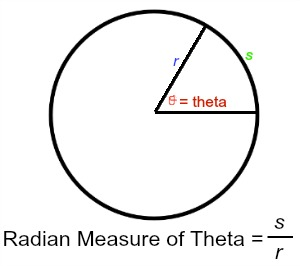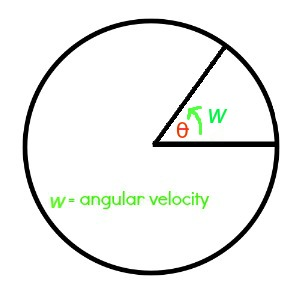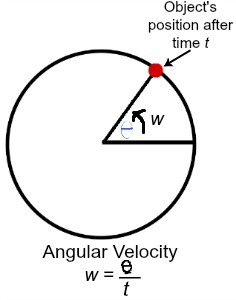# Angular Velocity: Definition, Formula & Examples

An error occurred trying to load this video.

Try refreshing the page, or contact customer support.

Coming up next: Average Velocity: Definition, Formula & Examples

### You're on a roll. Keep up the good work!

Replay
Your next lesson will play in 10 seconds
• 0:00 Linear Velocity
• 2:22 Angular Velocity
• 3:14 Angular Velocity Formulas
• 6:11 Lesson Summary

Want to watch this again later?

Timeline
Autoplay
Autoplay
Speed

#### Recommended Lessons and Courses for You

Lesson Transcript
Instructor: Laura Pennington

Laura has taught collegiate mathematics and holds a master's degree in pure mathematics.

Angular velocity applies to objects that move along a circular path. We will explore the definition of angular velocity and learn three different formulas we can use to calculate this type of velocity.

## Linear Velocity

Before we can get to angular velocity, we will first review linear velocity. Linear velocity applies to an object or particle that is moving in a straight line. It is the rate of change of the object's position with respect to time.One of the most common examples of linear velocity is your speed when you are driving down the road. Your speedometer gives your speed, or rate, in miles per hour. This is the rate of change of your position with respect to time, in other words, your speed is your linear velocity.

Linear velocity can be calculated using the formula v = s / t, where v = linear velocity, s = distance traveled, and t = time it takes to travel distance. For example, if I drove 120 miles in 2 hours, then to calculate my linear velocity, I'd plug s = 120 miles, and t = 2 hours into my linear velocity formula to get v = 120 / 2 = 60 miles per hour.

We have one more thing to review before getting to angular velocity, and that is radians. When we deal with angular velocity, we use the radian measure of an angle, so it is important that we are familiar with radian measure. The technical definition of radian measure is the length of the arc subtended by the angle, divided by the radius of the circle the angle is a part of, where subtended means to be opposite of the angle and to extend from one point on the circle to the other, both marked off by the angle. This tells us that an angle theta = s / r radians, where s = length of the arc corresponding to theta, and r = radius of the circle theta is a part of.Since most of us are comfortable with the degree measurement of angles, it is convenient that we can easily convert degree measure to radian measure by multiplying the degree measure by pi / 180. For example, a 45 degree angle has a radian measure 45 (pi / 180), which is equal to pi / 4 radians.

## Angular Velocity

Angular velocity is less common than linear velocity, because it only applies to objects that are moving along a circular path. For instance, a racecar on a circular track, a roulette ball on a roulette wheel, or a Ferris wheel, all have an angular velocity.The angular velocity of an object is the object's angular displacement with respect to time. When an object is traveling along a circular path, the central angle corresponding to the object's position on the circle is changing. The angular velocity, represented by w, is the rate of change of this angle with respect to time.For example, a Ferris wheel may be rotating pi / 6 radians every minute. Therefore, the Ferris wheel's angular velocity would be pi / 6 radians per minute.

## Angular Velocity Formulas

There are three formulas we can use to find angular velocity.

Option 1

The first comes straight from the definition. Angular velocity is the rate of change of the position angle of an object with respect to time, so w = theta / t, where w = angular velocity, theta = position angle, and t = time.To unlock this lesson you must be a Study.com Member.

### Register to view this lesson

Are you a student or a teacher?

### Unlock Your Education

#### See for yourself why 30 million people use Study.com

##### Become a Study.com member and start learning now.
Back
What teachers are saying about Study.com

### Earning College Credit

Did you know… We have over 160 college courses that prepare you to earn credit by exam that is accepted by over 1,500 colleges and universities. You can test out of the first two years of college and save thousands off your degree. Anyone can earn credit-by-exam regardless of age or education level.